# A transformer is a device for increasing or

• Slides: 13A transformer is a device for increasing or decreasing an a. c. voltage.Structure of TransformerCircuit Symbol for Transformer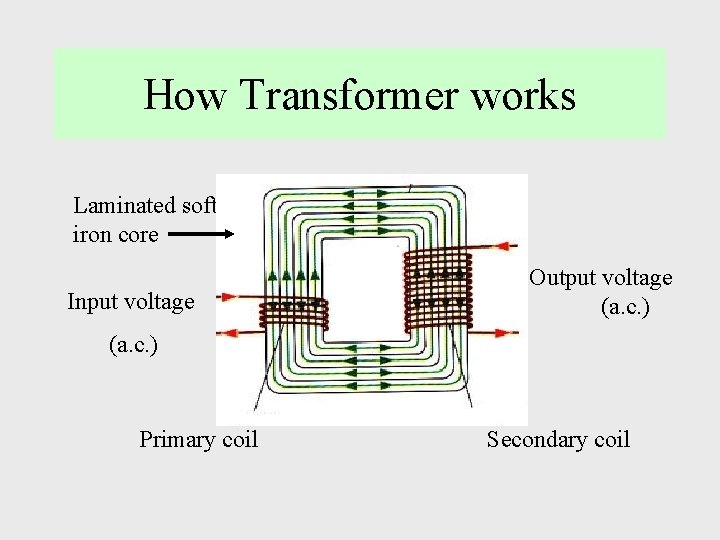How Transformer works Laminated soft iron core Input voltage Output voltage (a. c. ) Primary coil Secondary coil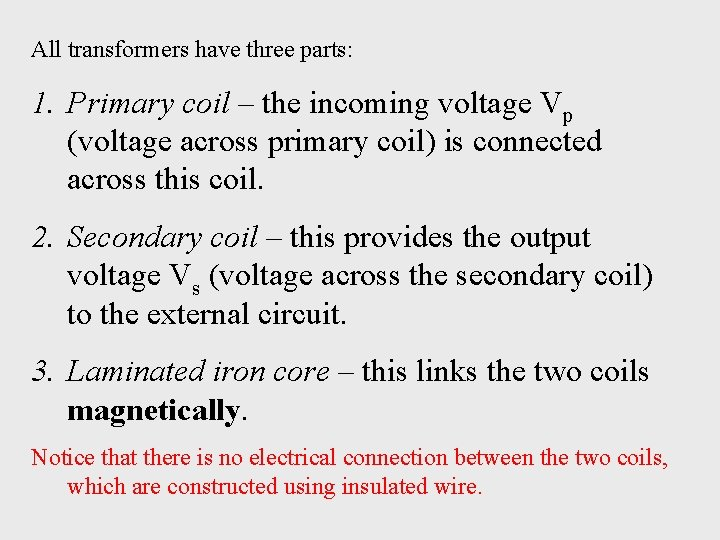All transformers have three parts: 1. Primary coil – the incoming voltage Vp (voltage across primary coil) is connected across this coil. 2. Secondary coil – this provides the output voltage Vs (voltage across the secondary coil) to the external circuit. 3. Laminated iron core – this links the two coils magnetically. Notice that there is no electrical connection between the two coils, which are constructed using insulated wire.Two Types of Transformer A step-up transformer increases the voltage there are more turns on the secondary than on the primary. A step-down transformer decreases the voltage - there are fewer turns on the secondary than on the primary. To step up the voltage by a factor of 10, there must be 10 times as many turns on the secondary coil as on the primary. The turns ratio tells us the factor by which the voltage will be changed.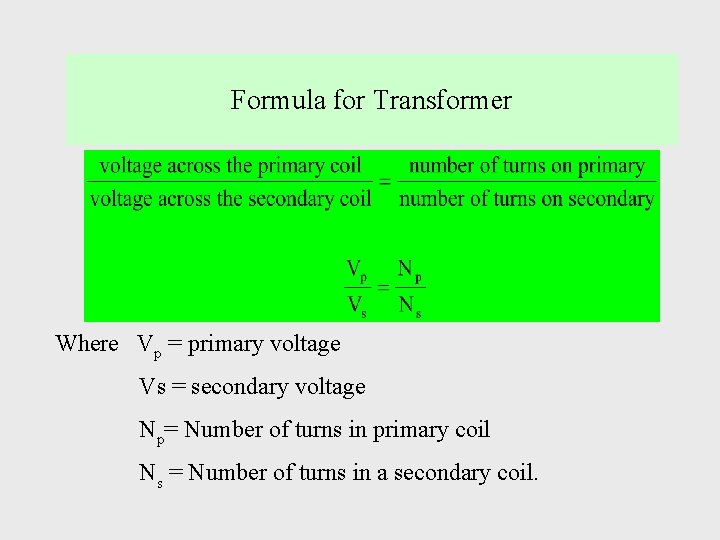Formula for Transformer Where Vp = primary voltage Vs = secondary voltage Np= Number of turns in primary coil Ns = Number of turns in a secondary coil.Worked example No. 1 The diagram shows a transformer. Calculate the voltage across the secondary coil of this transformer. Step-up transformer!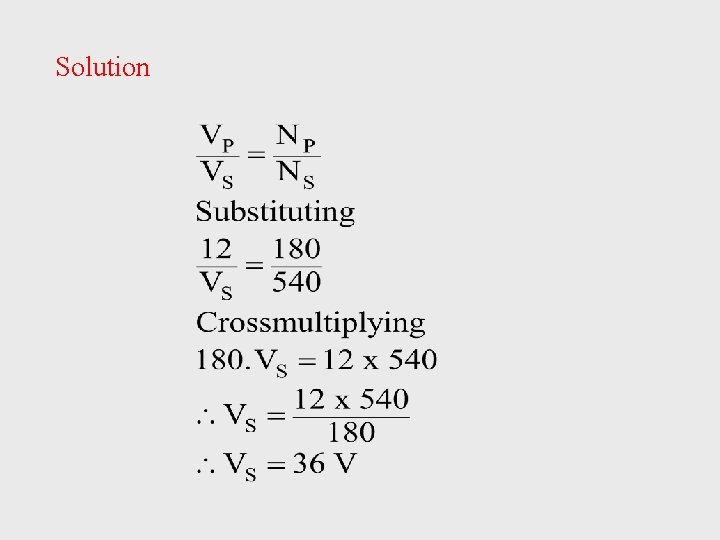SolutionWorked example No. 2 A transformer which has 1380 turns in its primary coil is to be used to convert the mains voltage of 230 V to operate a 6 V bulb. How many turns should the secondary coil of this transformer have? VP = 230 V NP = 1380 Obviously, a Step-down transformer!! VS = 6 V NS = ?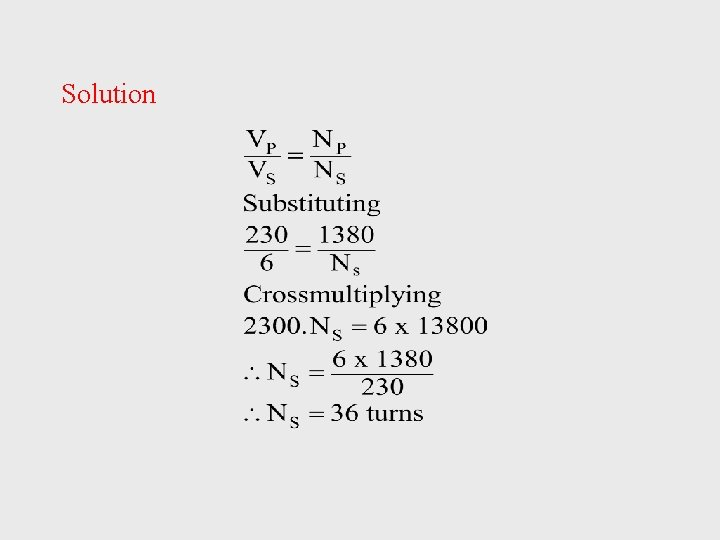Solution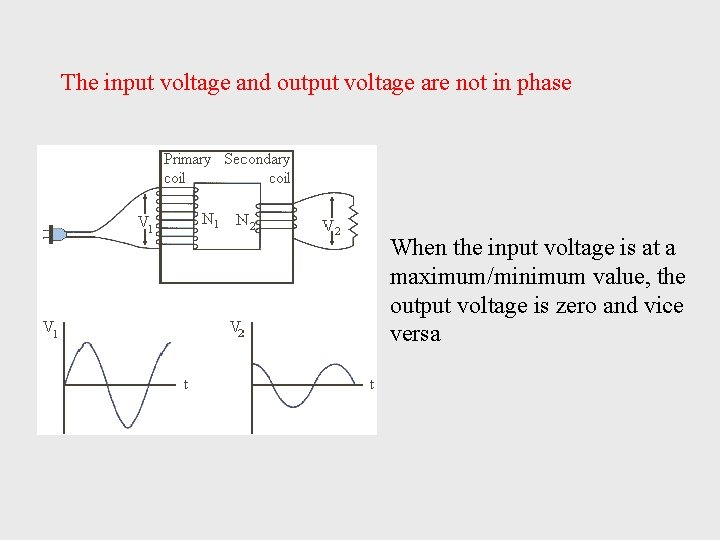The input voltage and output voltage are not in phase When the input voltage is at a maximum/minimum value, the output voltage is zero and vice versa